Most of the time they aren't set up that nicely.  You'll usually have to do a little dinking before something will drop out.

Look at this one: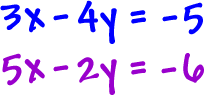If we just add straight down, nothing's going to drop out and we'll just get a mess.

But, check out the y guys: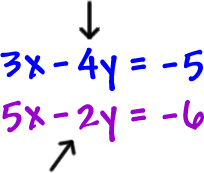If we could make this a +4y,
the
y's would drop out...

So, let's do it!  Remember that we can multiply an equation by a number...  So, let's multiply the second equation by a -2: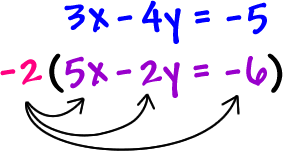Remember to hit each guy!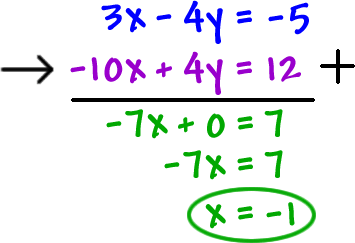Now, stick the x guy into either of the original equations.  I'm going to go for the first one: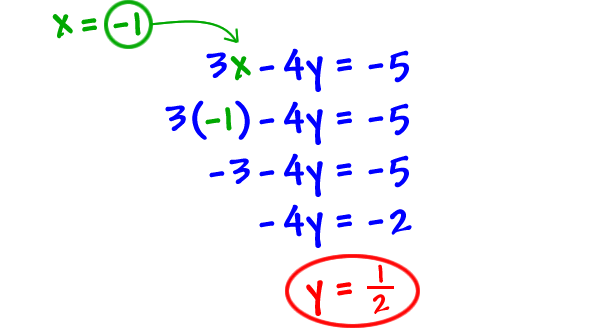The answer is (-1,)

Check it - and don't let that fraction freak you...  These things happen!